Function Repository Resource:

# TableToTrainingSet

Prepare rectangular data for use in the machine learning functions Classify, Predict and NetTrain

Contributed by: Jon McLoone
 ResourceFunction["TableToTrainingSet"][data,n] converts a matrix to a list of example→output pairs where the output is taken from column n. ResourceFunction["TableToTrainingSet"][assocs,key] converts associations to a list of example→output pairs where the output is labeled with key.

## Details and Options

The data can be lists, associations or a Dataset.

## Examples

### Basic Examples (2)

Prepare a matrix so that Classify can learn to predict column 3:

 In:=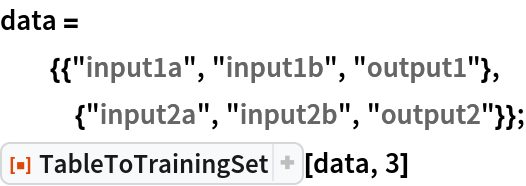Out=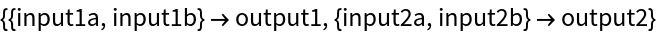In:=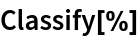Out=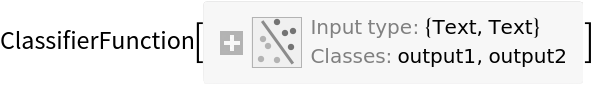Prepare an Association so that Predict can learn to predict the "output" values:

 In:=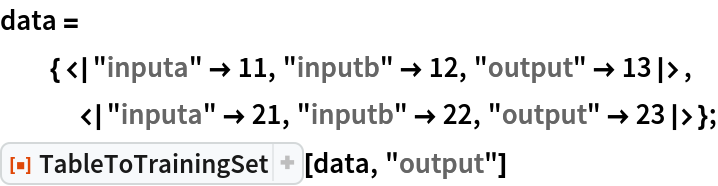Out=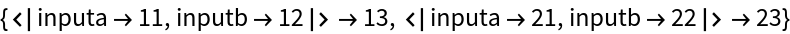In:=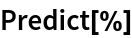Out=Jon McLoone

## Requirements

Wolfram Language 11.3 (March 2018) or above

## Version History

• 2.0.0 – 16 June 2020
• 1.0.0 – 07 March 2019